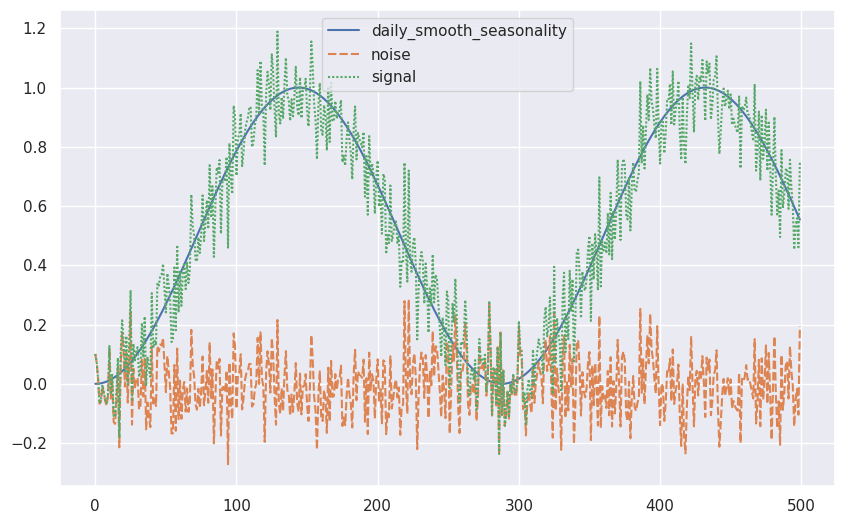# Synthetic Time Series¶

With a proper understanding of the DGP, we can build data generators around the DGP we choose.

## GluonTS¶

GluonTS is a python package for probabilistic time series modeling. It comes with a simple yet easy-to-use synthetic data generator. For example, to generate a time series of random Gaussian, we only need the following code1.

from gluonts.dataset.artificial import recipe as rcp
g_rg = rcp.RandomGaussian(stddev=2)
g_rp_series = rcp.evaluate(g_rg, 100)


For more complicated multivariate time series, we create recipes for our variables. We steal the example in the GluonTS tutorial.

from gluonts.dataset.artificial import recipe as rcp
import matplotlib.pyplot as plt
import seaborn as sns; sns.set()

daily_smooth_seasonality = rcp.SmoothSeasonality(period=288, phase=-72)
noise = rcp.RandomGaussian(stddev=0.1)
signal = daily_smooth_seasonality + noise

recipe = dict(
daily_smooth_seasonality=daily_smooth_seasonality, noise=noise, signal=signal
)
rec_eval = rcp.evaluate(recipe, 500)

fig, ax = plt.subplots(figsize=(10, 6.18))
sns.lineplot(rec_eval)1. Synthetic data generation. In: GluonTS documentation [Internet]. [cited 13 Nov 2022]. Available: https://ts.gluon.ai/stable/tutorials/data_manipulation/synthetic_data_generation.html

Contributors: LM